# FFTW3学习笔记2：FFTW（快速傅里叶变换）中文参考

## 一、 简介

  #include ... { fftw_complex *in, *out; fftw_plan p; ... in = (fftw_complex*) fftw_malloc(sizeof(fftw_complex) * N); out = (fftw_complex*) fftw_malloc(sizeof(fftw_complex) * N); // 输入数据in赋值 p = fftw_plan_dft_1d(N, in, out, FFTW_FORWARD, FFTW_ESTIMATE); fftw_execute(p); // 执行变换 ... fftw_destroy_plan(p); fftw_free(in); fftw_free(out); }

## 二、 一维复数据的DFT

1. 数据类型

fftw_complex默认由两个double组成，在内存中顺序排列，实部在 前，虚部在后，即typedef double fftw_complex。FFTW文档指出如果有一个支持C99标准的C编译器（如gcc），可以在#include <fftw3.h>前加入#include <complex.h>，这样一来fftw_complex就被定义为本机复数类型，而且与上述typedef二进制兼容（指内存排列），经 测试不能用在Windows下。C++有一个复数模板类complex<T>，在头文件<complex>下定义。C++标准委 员会最近同意该类的存储方式与C99二进制兼容，即顺序存储，实部在前，虚部在后（见报告WG21/N1388），该解决方案在所有主流标准库实现中都能正确工作。所以实际上可以用complex <double> 来代替fftw_complex，比如有一个复数数组complex<double> *x，则可以将其类型转换后作为参数传递给fftw：reinterpret_cast<fftw_complex*>(x)。测试如下：开 两个数组fftw_complex x1和complex<double> x2，然后赋相同值，在调试模式下可以看到它们的内存排列是相同的。complex<T>类数据赋值的方式不是很直接，必须采用无名对象方式x2[i] = complex <double>(1,2) 或成员函数方式x2[i].real(1);x2[i].imag(2);不能直接写x2[i].real=1;x2[i].imag=2。 fftw_complex赋值方式比较直接：x1[i]=1;x1[i]=2。最后，考虑到数据对齐（见后），最好使用 fftw_malloc分配内存，所以可以将其返回的指针转换为complex <double> *类型使用（比如赋值或读取等），变换时再将其转换为fftw_complex*。

2. 函数接口

 fftw_plan fftw_plan_dft_1d(int n, fftw_complex *in, fftw_complex *out, int sign, unsigned flags);

n为数据个数，可以为任意正整数，但如果为一些小因子的乘积计算起来可以更有效，不过即使n为素数算法仍然能够达到O(nlogn)的复杂度。FFTW对N=2a 3b 5c 7d 11e 13f的变换处理得最好，其中e+f=0/1，其它幂指数可以为任意值。

sign可以为正变换FFTW_FORWARD(-1)，也可以为逆变换FFTW_BACKWORD(+1)，实际上就是变换公式中指数项的符号。需注意FFTW的逆变换没有除以N，即数据正变换再反变换后是原始数据的N倍。

flags参数一般情况下为FFTW_MEASURE 或 FFTW_ESTIMATE。FFTW_MEASURE表示FFTW会先计算一些FFT并测量所用的时间，以便为大小为n的变换寻找最优的计算方法。依据 机器配置和变换的大小（n），这个过程耗费约数秒（时钟clock精度）。FFTW_ESTIMATE则相反，它直接构造一个合理的但可能是次最优的方 案。总体来说，如果你的程序需要进行大量相同大小的FFT，并且初始化时间不重要，可以使用FFTW_MEASURE，否则应使用 FFTW_ESTIMATE。FFTW_MEASURE模式下in和out数组中的值会被覆盖，所以该方式应该在用户初始化输入数据in之前完成。

  int length = 50000; fftw_complex* din = (fftw_complex *)fftw_malloc(sizeof(double)*length * 2); fftw_complex* dout = (fftw_complex *)fftw_malloc(sizeof(double)*length * 2); fftw_plan p = fftw_plan_dft_1d(length, din, din, FFTW_FORWARD, FFTW_MEASURE); fftw_execute(p); // 输入数据din赋值 // ... fftw_execute(p); // 读取变换结果 // ... fftw_destroy_plan(p); fftw_free(din); fftw_free(dout);

## 三、 多维复数据的DFT

  fftw_plan fftw_plan_dft_2d(int n0, int n1, fftw_complex *in, fftw_complex *out, int sign, unsigned flags); fftw_plan fftw_plan_dft_3d(int n0, int n1, int n2, fftw_complex *in, fftw_complex *out, int sign, unsigned flags); fftw_plan fftw_plan_dft(int rank, const int *n, fftw_complex *in, fftw_complex *out, int sign, unsigned flags);

## 四、 一维实数据的DFT

 fftw_plan fftw_plan_dft_r2c_1d(int n, double *in, fftw_complex *out, unsigned flags); fftw_plan fftw_plan_dft_c2r_1d(int n, fftw_complex *in, double *out, unsigned flags);

r2c版本：实输入数据，复Hermitian输出，正变换。

c2r版本：复Hermitian输入数据，实输出数据，逆变换。

n：逻辑长度，不必为物理长度。由于实数据的DFT具有 Hermitian对称性，所以只需要计算n/2+1（向下取整）个输出就可以了。比如对于r2c，输入in有n个数据，输出out有floor(n /2)＋1个数据。对于原位运算，in和out为同一数组（out须强制类型转换），所以其必须足够大以容纳所有数据，长度为2*(n/2+1)，in数 组的前n个数据为输入数据，后面的数据用来保存输出。

c2r逆变换在任何情况下（不管是否为原位运算）都破坏输入数组in，如果有必要可以通过在flags中加入FFTW_PRESERVE_INPUT来阻止，但这会损失一些性能，而其这个标志位目前在多维实DFT中已不被支持。

## 五、 多维实数据的DFT

  fftw_plan fftw_plan_dft_r2c_2d(int n0, int n1, double *in, fftw_complex *out, unsigned flags); fftw_plan fftw_plan_dft_r2c_3d(int n0, int n1, int n2, double *in, fftw_complex *out, unsigned flags); fftw_plan fftw_plan_dft_r2c(int rank, const int *n, double *in, fftw_complex *out, unsigned flags);

## 六、 更多实数据的DFT

  fftw_plan fftw_plan_r2r_1d(int n, double *in, double *out, fftw_r2r_kind kind, unsigned flags); fftw_plan fftw_plan_r2r_2d(int n0, int n1, double *in, double *out, fftw_r2r_kind kind0, fftw_r2r_kind kind1, unsigned flags); fftw_plan fftw_plan_r2r_3d(int n0, int n1, int n2, double *in, double *out, fftw_r2r_kind kind0, fftw_r2r_kind kind1, fftw_r2r_kind kind2, unsigned flags); fftw_plan fftw_plan_r2r(int rank, const int *n, double *in, double *out, const fftw_r2r_kind *kind, unsigned flags);

1. 半复数格式DFT（HalfComplex-format）

r0, r1, r2, ..., rn/2, i(n+1)/2-1, ..., i2, i1

2. DCT/DST

DCT可以认为是实偶对称数据DFT（REDFT,Real-Even DFT）, DST可以认为是实奇对称数据DFT（RODFT,Real-Odd DFT）。REDFTab和RODFTab中的a,b为数据移位标志（1表示移位），这些构成了DCT和DST的I－IV类，比较常用的为DCT-II，FFTW支持所有这些类型的变换。

 FFTW_REDFT00 (DCT-I): even around j=0 and even around j=n-1. FFTW_REDFT10 (DCT-II, the DCT): even around j=-0.5 and even around j=n-0.5. FFTW_REDFT01 (DCT-III, the IDCT): even around j=0 and odd around j=n. FFTW_REDFT11 (DCT-IV): even around j=-0.5 and odd around j=n-0.5. FFTW_RODFT00 (DST-I): odd around j=-1 and odd around j=n. FFTW_RODFT10 (DST-II): odd around j=-0.5 and odd around j=n-0.5. FFTW_RODFT01 (DST-III): odd around j=-1 and even around j=n-1. FFTW_RODFT11 (DST-IV): odd around j=-0.5 and even around j=n-0.5.

R*DFT01和R*DFT10要比R*DFT11稍微快一些，尤其对于奇数长度数据；而R*DFT00则要慢一些，尤其对于奇数长度数据。

1. 将out_matlab乘以√6×√6（即√N×√N）；

2. 再将上一步得到的out_matlab的第一行和第一列都乘以√2，因此第一个元素（即左上角的元素）要乘以2。

3. 其它

fftw_malloc考虑了数据对齐，以便使 用SIMD指令加速，所以最好不要用C函数malloc替代，而且不要将fftw_malloc、fftw_free和malloc、free、 delete等混用。尽量使用fftw_malloc分配数组，而不是下面的固定数组，因为固定数组是在栈上分配的，而栈空间较小；还因为这种方式没有考 虑数据对齐，不便应用SIMD指令。

 fftw_complex data[N0][N1][N2]; fftw_plan plan; ... plan = fftw_plan_dft_3d(N0, N1, N2, &data, &data, FFTW_FORWARD, FFTW_ESTIMATE); ...

 fftw_complex *a_good_array; a_good_array = (fftw_complex*) fftw_malloc(5*12*27* sizeof(fftw_complex)); fftw_complex ***a_bad_array; /* another way to make a 5x12x27 array */ a_bad_array = (fftw_complex ***) malloc(5 * sizeof(fftw_complex **));

## 七、 函数参考

1. 复数DFT

  fftw_plan fftw_plan_dft_1d(int n, fftw_complex *in, fftw_complex *out, int sign, unsigned flags); fftw_plan fftw_plan_dft_2d(int n0, int n1, fftw_complex *in, fftw_complex *out, int sign, unsigned flags); fftw_plan fftw_plan_dft_3d(int n0, int n1, int n2, fftw_complex *in, fftw_complex *out, int sign, unsigned flags); fftw_plan fftw_plan_dft(int rank, const int *n, fftw_complex *in, fftw_complex *out, int sign, unsigned flags);

2. 实数DFT

  fftw_plan fftw_plan_dft_r2c_1d(int n, double *in, fftw_complex *out, unsigned flags); fftw_plan fftw_plan_dft_r2c_2d(int n0, int n1, double *in, fftw_complex *out, unsigned flags); fftw_plan fftw_plan_dft_r2c_3d(int n0, int n1, int n2, double *in, fftw_complex *out, unsigned flags); fftw_plan fftw_plan_dft_r2c(int rank, const int *n, double *in, fftw_complex *out, unsigned flags);

3. 实数－实数变换

  fftw_plan fftw_plan_r2r_1d(int n, double *in, double *out, fftw_r2r_kind kind, unsigned flags); fftw_plan fftw_plan_r2r_2d(int n0, int n1, double *in, double *out, fftw_r2r_kind kind0, fftw_r2r_kind kind1, unsigned flags); fftw_plan fftw_plan_r2r_3d(int n0, int n1, int n2, double *in, double *out, fftw_r2r_kind kind0, fftw_r2r_kind kind1, fftw_r2r_kind kind2, unsigned flags); fftw_plan fftw_plan_r2r(int rank, const int *n, double *in, double *out, const fftw_r2r_kind *kind, unsigned flags);

4. 实数－实数变换类型

 FFTW_R2HC computes a real-input DFT with output in halfcomplex format, i.e. real and imaginary parts for a transform of size n stored as:r0, r1, r2, ..., rn/2, i(n+1)/2-1, ..., i2, i1 (Logical N=n, inverse is FFTW_HC2R.) FFTW_HC2R computes the reverse of FFTW_R2HC, above. (Logical N=n, inverse is FFTW_R2HC.) FFTW_DHT computes a discrete Hartley transform. (Logical N=n, inverse is FFTW_DHT.) FFTW_REDFT00 computes an REDFT00 transform, i.e. a DCT-I. (Logical N=2*(n-1), inverse is FFTW_REDFT00.) FFTW_REDFT10 computes an REDFT10 transform, i.e. a DCT-II (sometimes called the DCT). (Logical N=2*n, inverse is FFTW_REDFT01.) FFTW_REDFT01 computes an REDFT01 transform, i.e. a DCT-III (sometimes called the IDCT, being the inverse of DCT-II). (Logical N=2*n, inverse is FFTW_REDFT=10.) FFTW_REDFT11 computes an REDFT11 transform, i.e. a DCT-IV. (Logical N=2*n, inverse is FFTW_REDFT11.) FFTW_RODFT00 computes an RODFT00 transform, i.e. a DST-I. (Logical N=2*(n+1), inverse is FFTW_RODFT00.) FFTW_RODFT10 computes an RODFT10 transform, i.e. a DST-II. (Logical N=2*n, inverse is FFTW_RODFT01.) FFTW_RODFT01 computes an RODFT01 transform, i.e. a DST-III. (Logical N=2*n, inverse is FFTW_RODFT=10.) FFTW_RODFT11 computes an RODFT11 transform, i.e. a DST-IV. (Logical N=2*n, inverse is FFTW_RODFT11.)

## 八、 其它

1. 数据类型

FFTW有三个版本的数据类型：double、float和long double，使用方法如下：

• 链接对应的库（比如libfftw3-3、libfftw3f-3、或ibfftw3l-3）
• 包含同样的头文件fftw3.h
• 将所有以小写"fftw_"开头的名字替换为"fftwf_"（float版本）或"fftwl_"（long double版本）。比如将fftw_complex替换为fftwf_complex，将fftw_execute替换为fftwf_execute等。
• 所有以大写"FFTW_"开头的名字不变
• 将函数参数中的double替换为float或long double

2. 用同一个fftw_plan执行多个数据的变换

• 输入/输出数据大小相等。
• 变换类型、是否原位运算不变。
• 对split数组（指实虚部分开），实部和虚部的分割方式与方案创建时相同。
• 数组的对齐方式相同。如果都是用fftw_malloc分配的则此项条件满足，除非使用 FFTW_UNALIGNED标志。

  void fftw_execute_dft( const fftw_plan p, fftw_complex *in, fftw_complex *out); void fftw_execute_split_dft( const fftw_plan p, double *ri, double *ii, double *ro, double *io); void fftw_execute_dft_r2c( const fftw_plan p, double *in, fftw_complex *out); void fftw_execute_split_dft_r2c( const fftw_plan p, double *in, double *ro, double *io); void fftw_execute_dft_c2r( const fftw_plan p, fftw_complex *in, double *out); void fftw_execute_split_dft_c2r( const fftw_plan p, double *ri, double *ii, double *out); void fftw_execute_r2r( const fftw_plan p, double *in, double *out);

3. 多线程FFTW

FFTW可以多线程执行，但是多线程存在线程同步问题，这可能会降低性能。所以除非问题规模非常大，一般并不能从多线程中获益。

4. FFTW变换公式

 一维复DFT正变换一维复DFT逆变换一维实DFT正变换（Yk = Yn-k * ）一维实DFT逆变换REDFT00 (DCT-I)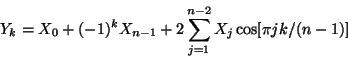REDFT10 (DCT-II)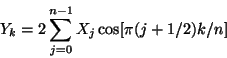REDFT01 (DCT-III)REDFT11 (DCT-IV)RODFT00 (DST-I)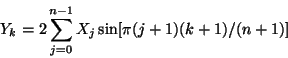RODFT10 (DST-II)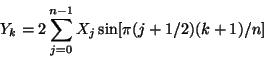RODFT01 (DST-III)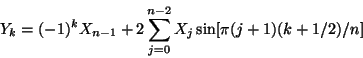RODFT11 (DST-IV)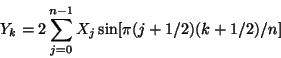1d Discrete Hartley Transforms (DHTs)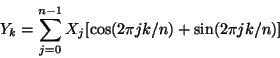posted @ 2018-08-02 15:28  爱国呐  阅读(9621)  评论(0编辑  收藏